# Ammeter Design

## DC Electric Circuits

• #### Question 1

What would happen to this meter movement, if connected directly to a 6-volt battery?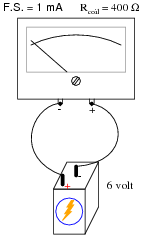• #### Question 2

We know that connecting a sensitive meter movement directly in series with a high-current circuit is a Bad Thing. So, I want you to determine what other component(s) must be connected to the meter movement to limit the current through its coil, so that connecting the circuit in series with a 1-amp circuit results in the meter’s needle moving exactly to the full-scale position.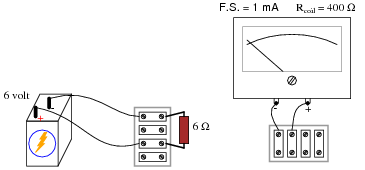In your diagram, show both the extra component(s) and the manner in which the meter assembly will be connected to the battery/resistor circuit to measure current.

• #### Question 3

Determine the measurement range of this ammeter: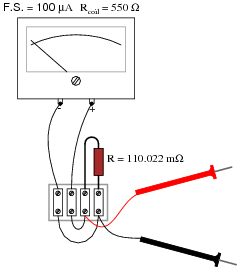• #### Question 4

What will happen to the function of this ammeter circuit, if the wire marked in the illustration were to fail open?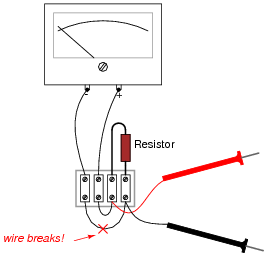• #### Question 5

What will happen to the function of this ammeter circuit, if its resistor were to fail open?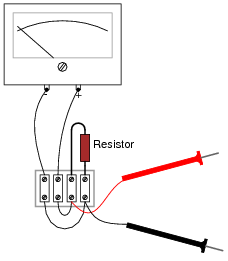• #### Question 6

Shown here is an ammeter circuit with a special type of selector switch, called a make-before-break selector: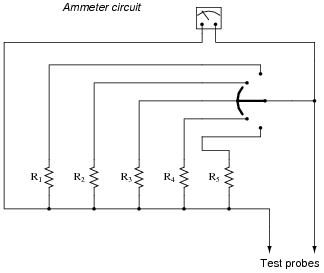This special kind of selector switch is important to have in an ammeter circuit such as the one shown above. If we were to build such a similar ammeter using a normal (break-before-make) selector switch, the meter would be susceptible to damage during normal use:Explain why the first circuit design is superior to the second, and what form of use would prove damaging to the second design (but not to the first).

• #### Question 7

Ideally, should an ammeter have a very low input resistance, or a very high input resistance (input resistance being the amount of electrical resistance intrinsic to the meter, as measured between its test leads)? Explain your answer.

• #### Question 8

For any given range of current measurement, what design parameter(s) of an electromechanical ammeter influence its input resistance? In other words, to approach the “ideal” input resistance of an ammeter, for any given range, what component values are optimum?

• #### Question 9

Shunt resistors are often used as current-measuring devices, in that they are designed to drop very precise amounts of voltage as large electric currents pass through them. By measuring the amount of voltage dropped by a shunt resistor, you will be able to determine the amount of current going through it: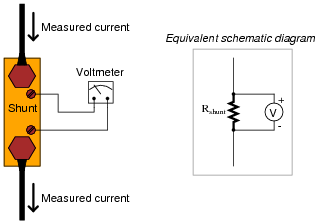Suppose that a shunt resistance is labeled with the following rating: 150 A , 50 mV. What is the resistance of this shunt, in ohms? Express your answer in metric notation, scientific notation, and plain decimal notation.

• #### Question 10

Shunt resistors used for precision current measurement always have four terminals for the electrical connections, even though normal resistors only have two: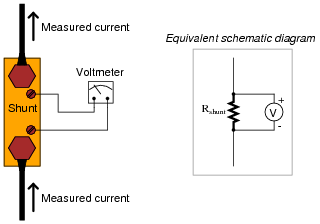Explain what would be wrong with connecting the voltmeter movement directly to the same two terminals conducting high current through the shunt resistor, like this: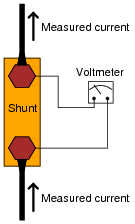• #### Question 11

Shunt resistors, being very low in resistance, are usually made from relatively large masses of metal. Their precise resistance is calibrated through a process known as trimming, where a technician takes a metal file and “trims” metal from the shunt conductor until the resistance reaches its correct value. This, of course, only works if the shunt resistor is intentionally manufactured with a resistance that is too low. Like the old carpenter’s joke goes, “I cut the board twice and it’s still too short!”

Being that shunt resistors have such incredibly low resistance values, how do we measure the resistance of a shunt with high accuracy during the “trimming” process? The resistance of a shunt is far too low for an average handheld or even benchtop ohmmeter to measure with precision, and specialized low-resistance ohmmeters such as the Kelvin Double Bridge are quite expensive. If you were given the task of trimming a shunt resistor for use in an ammeter, and you only possessed average pieces of test equipment, how could you do it?

• #### Question 12

An important step in building any analog voltmeter or ammeter is to accurately determine the coil resistance of the meter movement. In electrical metrology, it is often easier to obtain extremely precise (“standard”) resistance values than it is to obtain equally precise voltage or current measurements. One technique that may be used to determine the coil resistance of a meter movement without need to accurately measure voltage or current is as follows.

First, connect a decade box type of variable resistance in series with a regulated DC power supply, then to the meter movement to be tested. Adjust the decade box’s resistance so that the meter movement moves to some precise point on its scale, preferably the full-scale (100%) mark. Record the decade box’s resistance setting as R1:Then, connect a known resistance in parallel with the meter movement’s terminals. This resistance will be known as Rs, the shunt resistance. The meter movement deflection will decrease when you do this. Re-adjust the decade box’s resistance until the meter movement deflection returns to its former place. Record the decade box’s resistance setting as R2:The meter movement’s coil resistance (Rcoil) may be calculated following this formula:

 Rcoil = Rs R2 (R1 − R2)

Your task is to show where this formula comes from, deriving it from Ohm’s Law and whatever other equations you may be familiar with for circuit analysis.

Hint: in both cases (decade box set to R1 and set to R2), the voltage across the meter movement’s coil resistance is the same, the current through the meter movement is the same, and the power supply voltage is the same.

• #### Question 13

 Don’t just sit there! Build something!!

Learning to mathematically analyze circuits requires much study and practice. Typically, students practice by working through lots of sample problems and checking their answers against those provided by the textbook or the instructor. While this is good, there is a much better way.

You will learn much more by actually building and analyzing real circuits, letting your test equipment provide the “answers” instead of a book or another person. For successful circuit-building exercises, follow these steps:

1. Carefully measure and record all component values prior to circuit construction.
2. Draw the schematic diagram for the circuit to be analyzed.
3. Carefully build this circuit on a breadboard or other convenient medium.
4. Check the accuracy of the circuit’s construction, following each wire to each connection point, and verifying these elements one-by-one on the diagram.
5. Mathematically analyze the circuit, solving for all values of voltage, current, etc.
6. Carefully measure those quantities, to verify the accuracy of your analysis.
7. If there are any substantial errors (greater than a few percent), carefully check your circuit’s construction against the diagram, then carefully re-calculate the values and re-measure.

Avoid very high and very low resistor values, to avoid measurement errors caused by meter “loading”. I recommend resistors between 1 kΩ and 100 kΩ, unless, of course, the purpose of the circuit is to illustrate the effects of meter loading!

One way you can save time and reduce the possibility of error is to begin with a very simple circuit and incrementally add components to increase its complexity after each analysis, rather than building a whole new circuit for each practice problem. Another time-saving technique is to re-use the same components in a variety of different circuit configurations. This way, you won’t have to measure any component’s value more than once.